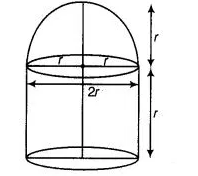# A building is in the form of a cylinder surmounted

Question:

A building is in the form of a cylinder surmounted by a hemispherical vaulted dome and contains $41 \frac{19}{21} \mathrm{~m}^{3}$ of air. If the

internal diameter of dome is equal to its total height above the floor, find the height of the building?

Solution:

Let total height of the building = Internal diameter of the dome = 2r m$\therefore \quad$ Radius of building (or dome) $=\frac{2 r}{2}=r \mathrm{~m}$

Height of cylinder $=2 r-r=r \mathrm{~m}$

$\therefore \quad$ Volume of the cylinder $=\pi r^{2}(r)=\pi r^{3} \mathrm{~m}^{3}$

and volume of hemispherical dome cylinder $=\frac{2}{3} \pi r^{3} \mathrm{~m}^{3}$

$\therefore$ Total volume of the building $=$ Volume of the cylinder $+$ Volume of hemispherical dome

$=\left(\pi r^{3}+\frac{2}{3} \pi r^{3}\right) m^{3}=\frac{5}{3} \pi r^{3} m^{3}$

According to the question,

Volume of the building = Volume of the air

$=\left(\pi r^{3}+\frac{2}{3} \pi r^{3}\right) \mathrm{m}^{3}=\frac{5}{3} \pi r^{3} \mathrm{~m}^{3}$

According to the question,

Volume of the building $=$ Volume of the air

$\Rightarrow$ $\frac{5}{3} \pi r^{3}=41 \frac{19}{21}$

$\Rightarrow$ $\frac{5}{3} \pi r^{3}=\frac{880}{21}$

$\Rightarrow$ $r^{3}=\frac{880 \times 7 \times 3}{21 \times 22 \times 5}=\frac{40 \times 21}{21 \times 5}=8$

$\Rightarrow$ $r^{3}=8 \Rightarrow r=2$

$\therefore$ Height of the building $=2 r=2 \times 2=4 \mathrm{~m}$